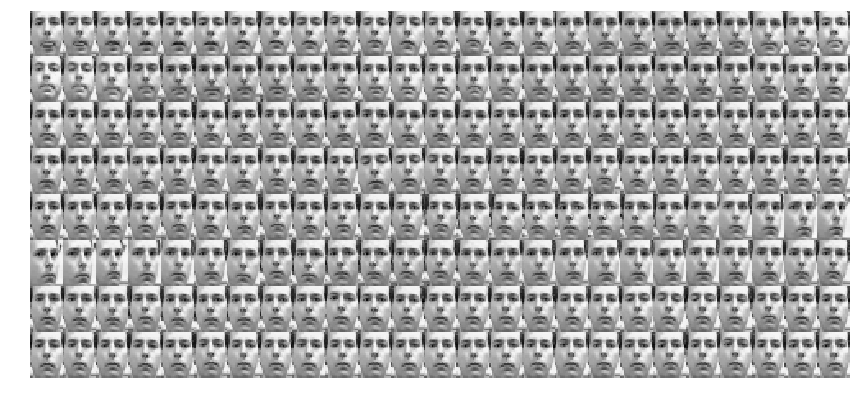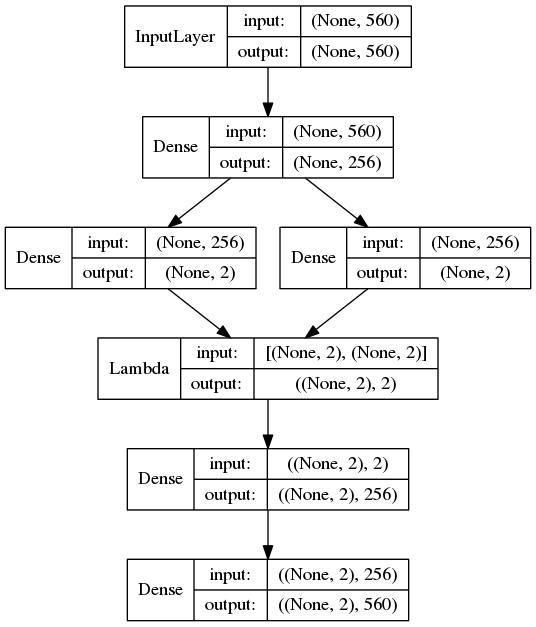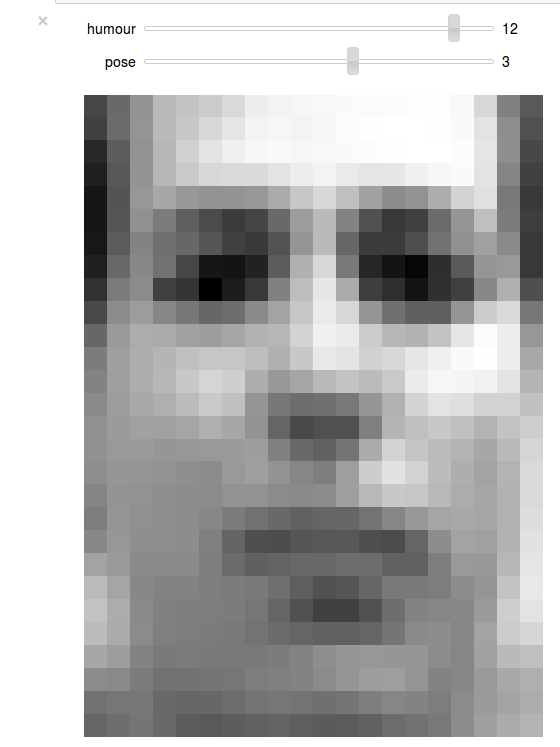In this post, I’ll demo variational auto-encoders [Kingma et al. 2014] on the “Frey faces” dataset, using the keras deep-learning Python library.

## Some formal preliminaries

A well-known thermodynamic variational bound on surprise goes as follows:

\begin{equation} \begin{split} -\log p_G(x) = F_G(x) = F^R_G(x) - D_{KL}(p_R(.|x)||P_G(.|x)) \le F^R_G(x), \end{split} \end{equation} where

• $x$: Examplar datavector (visible layer).
• $z$: Hidden / latent variable (these are the ‘causes’ of the datavectors).
• $G$: Generative model, with density $z \sim p_G(.|x)$, parametrized by a tensor of weights $W^G$ (we’ll use a neural network)
• $R$: Recognition model, with density $z \sim p_R(.|x)$, parametrized by a tensor of weights $W^R$ (we’ill use a NN).
• $D_{KL}(q||p)$ is the Kullback-Leibler divergence between probability densities $p$ and $q$, defined by \begin{equation} D_{KL}(q||p) := \sum_{z}q(z)\log(q(z)/p(z)) \end{equation}

• $F_G(x)$: Helmhost free-energy f a fictive thermodynamic system with macrostate energy levels $(E_G(z,x))_z$ with $E_G(z,x) := -\log(p_G(z,x))$, and partition function $p_G(x)$.
• $F^R_G(x)$ is the variational Helmholtz free-energy from $G$ to $R$, defined by \begin{equation} F_G^R(x) := \langle -\log(p_G(., x)) \rangle_{P_R(.|x)} - \mathcal H(P_R(.|x)), \end{equation} with \begin{equation} \mathcal H(p_R(.|x)) := -\sum_{z}p_R(z|x))\log(p_R(z|x)), \end{equation} the entropy of $p_R(.|x)$.

Problem: How do we sample from the recognition density $p_R(.|x)$ in such a way that the sampling process is differentiable w.r.t the weights of the recognition network $W^R$ ?

Solution: The reparametrization trick!

The solution proposed in [Kingma et al. 2014] is to use a reparametrization trick:

• Choose $\epsilon \sim p_{\text{noise}}$ (noise distribution, independent of $W^R$! )
• Set $z = g(W^{R}, x, \epsilon)$, where $g$ is an appropriate class $\mathcal C^1$ function.

$\implies$ a sample $z \sim p_R(.|x)$, from the correct posterior

## The code

Dependencies: We’ll need the following python libraries to get things running:

• Numpy / Scipy (install everything via anaconda)
• keras
• Theano or Tensforflow (as backend for Keras)

A bit of setup

Now, let’s load the dataset

… and split data into train / validation folds

Visualize some examples from the datasetBuild forward model (encoding)

Sample from latent space

Build backward model (decoding)

Build the autoencderTrain the autoencoder (or reload a previously trained one)

Separate encoder from input to latent space

Generator from latent to input space

Display a 2D manifold of the faces. In this example we found that the each dimension of the hidden variable z was encoding for socially meaningful things like humour / expression & pose